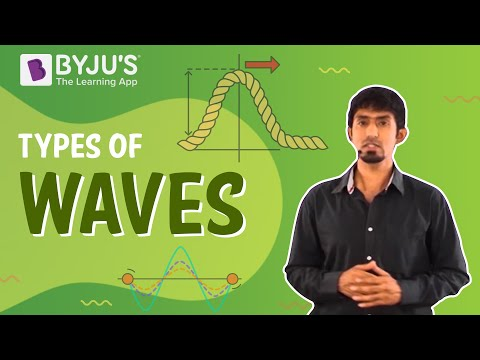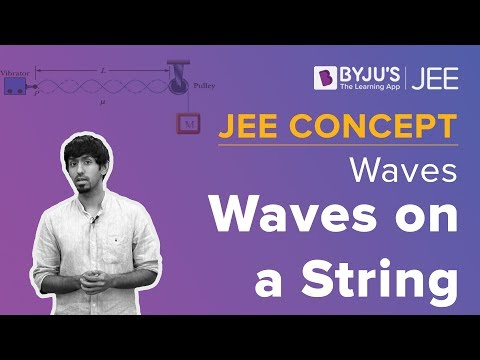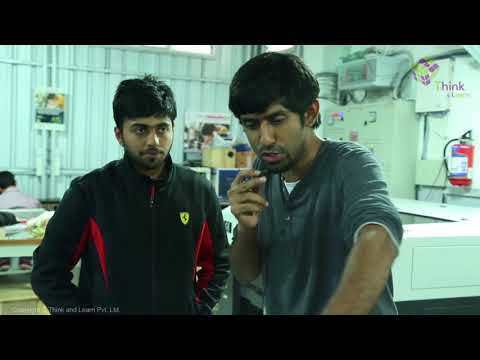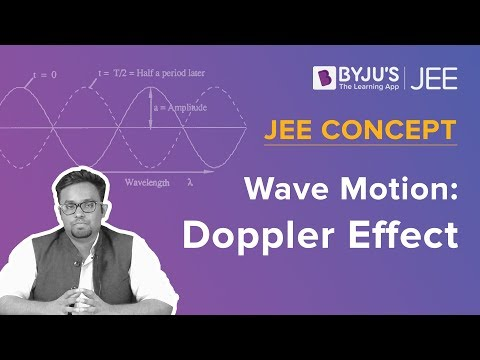# CBSE Class 11 Physics Notes Chapter 15 Waves

According to the CBSE Syllabus 2023-24, this chapter has been renumbered as Chapter 14.

## What are the types of waves?

We can witness four types of waves. They are:

• Mechanical Waves- can exist in material media and follow Newton’s laws.
• Transverse waves are referred to those whose particles oscillate in a perpendicular motion of the direction of propagation of the wave
• Longitudinal waves are referred to those whose particles oscillate along the way of the propagation of the wave
• When the waves move from one point of the medium to another is called a progressive waveAlso See: Wave Motion

### Wavelength of a wave

In the case of a progressive wave, the distance between two points in the same phase at that particular time period is known as the wavelength of a wave. The distance is twice the number of two consecutive nodes and antinodes.

### Time Period of oscillation

When an element of a medium takes time to move through one complete oscillation then it is called a time period.

### Principle of superposition of waves

In a medium when multiple waves transverse simultaneously, the displacement is the algebraic sum of the displacements due to each wave. This phenomenon is referred to as the principle of superposition.

### Standing waves

When two identical waves moving in opposite directions interfere, it results in a standing wave. These waves are characterized by zero displacement locations which are fixed and are called nodes and locations of maximum displacements called antinodes.

## Doppler Effect

The change in the frequency of a wave when the source or the observer or both are moving relative to the medium. This phenomenon is used in different scientific aspects such as planetary science wherein astronomers depend on this effect to identify planets exterior to the solar system. Doppler Effect is an increase (or decrease) in the frequency of sound, light, or other waves as the source and observer move towards (or away from) each other.

 Also Access NCERT Solutions for Class 11 Physics Chapter 15 NCERT Exemplar for Class 11 Physics Chapter 15

#### For more information on Standing Wave and Doppler Effect, watch the below videos### Important Questions

1. A string of mass 3 kg experiences a tension of 300 N. The stretched string’s length is 20 m. Calculate the time taken by the disturbance to reach the other end when one end is struck with a transverse jerk.
2. From a tower of height 400 m, a stone is dropped from the top into the water of the pond nearby. Calculate when the splash is heard at the top and the speed of sound in air is 340m/s (g=9.8 m/s2)
3. The length of the steel wire is 14 m and the mass is 3 kg. Find out the tension in the wire where the speed of the transverse wave on the wire equals the speed of sound in air at a temperature of 30 degrees= 353 m/s

 Doppler Effect Wavelength of Light

## Frequently Asked Questions on CBSE Class 11 Physics Notes Chapter 15 Waves

Q1

### What is a travelling wave?

A wave in which the positions of maximum and minimum amplitude travel through the medium is known as a travelling wave.

Q2

### What is meant by the superposition of wave?

The superposition principle states that when two or more waves overlap in space, the resultant disturbance is equal to the algebraic sum of the individual disturbances.

Q3

### What is meant by time period?

A time period is the time taken for one complete cycle of vibration to pass a given point.